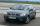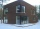Train from Prague

First train from Prague started at 8:00 hour at 40 kilometers per hour. Train from Ostrava started at 9:20 at 80 km per hour. In how many hours and how far from cities with trains meet if the distance of cities is 400 km.

Result

t = 12:13 hp =  168.889 km
o =  231.111 km

Solution:

p+o = 400
p = 40(t-8)
o = 80(t-(9+20/60))

o+p = 400
p-40t = -320
60o-4800t = -44800

o = 20809 ≈ 231.111111
p = 15209 ≈ 168.888889
t = 1109 ≈ 12.222222

Calculated by our linear equations calculator.

Leave us a comment of example and its solution (i.e. if it is still somewhat unclear...):Be the first to comment!To solve this example are needed these knowledge from mathematics:

Do you have a system of equations and looking for calculator system of linear equations? Do you want to convert length units?

Next similar examples:

1. Train from BrnoFirst train started from Brno at 8 am at 60 km per hour. Second train started from Opava at 7 o'clock at the speed of 40 kilometers per hour. In how many hours and how far from cities trains meet when the distance of cities is 200 kilometers.
2. Cars 6At 9:00 am two cars started from the same town and traveled at a rate of 35 miles per hour and the other car traveled at a rate of 40 miles per hour. After how many hours will the cars be 30 miles apart?
3. StormSo far, a storm has traveled 35 miles in 1/2 hour in direction straight to observer. If it is currently 5:00 p. m. and the storm is 105 miles away from you, at what time will the storm reach you? Explain how you solved the problem.
4. Round-tripA woman works at a law firm in city A which is about 50 miles from city B. She must go to the law library in city B to get a document. Find how long it takes her to drive​ round-trip if she averages 40 mph.
5. Freight and passenger carThe truck starts at 8 pm at 30 km/h. Passenger car starts at 8 pm at 40 km/h. Passenger arrives in the destination city 1 hour and 45 min earlier. What is the distance between the city of departure and destination city?
6. Jane plantsJane plants flowers in the garden. If she planted 12 every hour instead of 9 flowers, she would finish with the job an hour earlier. How many flowers does she plant?
7. Three brothersThe three brothers have a total of 42 years. Jan is five years younger than Peter and Peter is 2 years younger than Michael. How many years has each of them?
8. Waiting roomIn the waiting room are people and flies. Together they have 15 heads and 50 legs (fly has 6 legs). How many people and flies are in the waiting room?
9. MushroomsEva and Jane collected 114 mushrooms together. Eve found twice as much as Jane. How many mushrooms found each of them?
10. Grandfather and grandmotherThe old mother is 5 years younger than the old father. Together they are 153 years old. How many years has each of them?
11. Hotel roomsIn the 45 rooms, there were 169 guests, some rooms were three-bedrooms and some five-bedrooms. How many rooms were?
12. TreesAlong the road were planted 250 trees of two types. Cherry for 60 CZK apiece and apple 50 CZK apiece. The entire plantation cost 12,800 CZK. How many was cherries and apples?
13. TheatroTheatrical performance was attended by 480 spectators. Women were in the audience 40 more than men and children 60 less than half of adult spectators. How many men, women and children attended a theater performance?
14. Equations - simpleSolve system of linear equations: x-2y=6 3x+2y=4
15. Ages 2A man's age is 4 times his son's age. After 5 years he will be just twice his son's age, find their ages.
16. Bob traveledBob traveled 20 meters to Dave's house. He arrived in 3 minutes. What was his average speed for the trip.
17. EquationsSolve following system of equations: 6(x+7)+4(y-5)=12 2(x+y)-3(-2x+4y)=-44